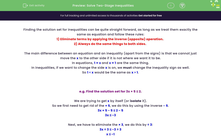# Solve Two-Stage Inequalities

In this worksheet, students will find solution sets for inequalities with two elements by applying inverse operations to isolate the target variable.Key stage:  KS 4

Year:  GCSE

GCSE Subjects:   Maths

GCSE Boards:   AQA, Eduqas, OCR, Pearson Edexcel,

Curriculum topic:   Algebra

Curriculum subtopic:   Solving Equations and Inequalities Algebraic Equations

Popular topics:   Algebra worksheets

Difficulty level:#### Worksheet Overview

Finding the solution set for inequalities can be quite straightforward, as long as we treat them exactly the same as equations and follow these rules:

1) Eliminate terms by applying the inverse (opposite) operation.

2) Always do the same things to both sides.

The main difference between an equation and an inequality (apart from the signs) is that we cannot just move the x to the other side if it is not where we want it to be.

In equations, 1 = x and x = 1 are the same thing.

In inequalities, if we want to change the side x is on, we must change the inequality sign as well.

So 1 < x would be the same as x > 1.

e.g. Find the solution set for 3x + 5 ≤ 2.

We are trying to get x by itself (or isolate it).

So we first need to get rid of the + 5, we do this by using the inverse - 5.

3x + 5 - 5 ≤ 2 - 5

3x ≤ -3

Next, we have to eliminate the × 3, we do this by ÷ 3:

3x ÷ 3 ≤ -3 ÷ 3

x ≤ -1

e.g. Find the solution set for -2 > 3x + 4.

We are trying to get x by itself, so first we need to get rid of the + 5, we do this by using - 5:

-2 - 4 > 3x + 4 - 4

-6 > 3x

Next, we have to eliminate the × 3, we do this by using ÷ 3:

- 6 ÷ 3 > 3x ÷ 3

-2 > x

Lastly, this inequality has its x on the right and it needs to be on the left.

To manage this, we also have to switch the inequality:

x < -2

In this activity, we will find solution sets for inequalities with two elements by isolating our target term on the left-hand side or flipping the side and switching the inequality sign if our variable is on the left.

Some of the working out for these questions can be lengthy, so you may want to have a pen and paper handy so you can compare your own working to our answers.

### What is EdPlace?

We're your National Curriculum aligned online education content provider helping each child succeed in English, maths and science from year 1 to GCSE. With an EdPlace account you’ll be able to track and measure progress, helping each child achieve their best. We build confidence and attainment by personalising each child’s learning at a level that suits them.

Get started